# Arbitrage Calculator

## Arbitrage calculator

In addition to welcoming arbitrage bettors, Pinnacle also provides an Arbitrage Calculator to help bettors work out potential arbitrage betting opportunities.

Arbitrage betting is a risk-free approach to betting that guarantees a profit. It involves placing proportional bets on every possible outcome of an event (with different bookmakers) so regardless of what happens, you will make a profit.

If you enter the Odds for any two-way or three-way market in the Arbitrage Calculator above, it will work out if there is an arbitrage opportunity and tell you how much you need to stake in order to guarantee a profit.

There are many different ways to calculate profit achieved with arbitrage bets. This page explains how arbitrage betting works and what you will have to calculate, manually or using arbitrage calculator, every time you place surebets. At first, we will show you how to find arbitrage bets. On the example below we will also show you the easiest way to calculate profit by taking advantage of different odds offered by different bookmakers. In the end, we will show you how to distribute your invest into individual bets.

In the beginning finding surebets and calculating profit may seem like a lot of work, but after few calculations you will be able to find arbs and estimate their profit just by looking at the odds. Throughout these calculations decimal (European) odds will be used because they don’t require a lot of conversion. Don’t worry about betting odds since all bookmakers can display decimal, fractional or moneyline odds.

## Finding arbitrage bets

We look for an event with two possible outcomes (e.g. tennis match – either Djokovic wins or Nadal wins), because arbs in events like this are the easiest to find.

First, you have to find two sportsbooks that offer the highest odds for each player. Our arbitrage betting guide explains methods of finding arbs. In the example below one sportsbook (5Dimes) offers the highest odds for the favorite (Djokovic) and the other sportsbook (Pinnacle) offers the highest odds for the underdog (Nadal).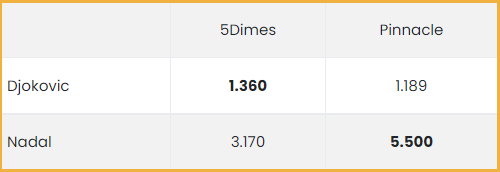If you are planning to find surebets yourself, the table below could be helpful. As said before, you have to find two sportsbooks that offer the highest odds on both outcomes. One bookie has to offer the highest odds on Outcome 1 and another bookie on Outcome 2. If you learn the numbers from the table, you will be able to estimate if there is an arbitrage opportunity simply by glancing at the odds at sportsbooks’ sites.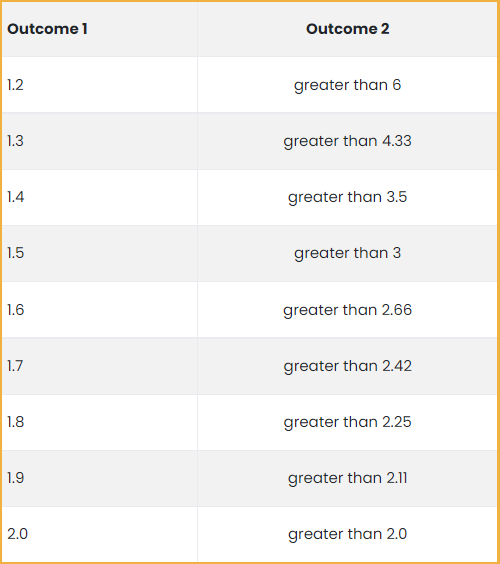## Calculating arbitrage percentage

After you have found bookies with the highest odds for particular match, you will have to calculate arbitrage percentage. The percentage represents the amount you have to invest in order to receive a total return of 100. In order to get arbitrage percentage you have to divide 1 with each set of odds and then add them together. The formula is: arbitrage percentage=(1/EU odds)*100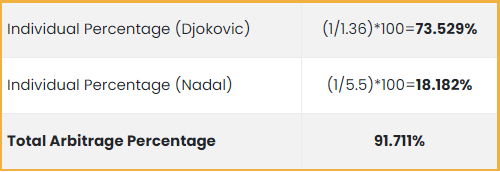If the sum of the percentages is less than 100% then you have an arb. For an individual bookmaker, arbitrage percentage is always greater than 100%. The spread above 100% is the bookmakers margin or return rate, the amount the bookie earns on offering bets for an event. That is why bookmakers always want to have balanced book. In the example above 5Dimes bookmaker expects to earn around 5% on bets (73.529%+31.546%=105.075%), while Pinnacle expects to earn around 2% on bets (84.104%+18.182%=102.286%). Usual bookmaker’s margin (return rate) is around 5%.

In arbitrage betting the idea is to find the odds at different sportsbooks, where the arbitrage percentage is below 100%. This means that the sportsbooks disagree on the chances of the outcome or have accepted too much money on one outcome of the event. This mismatch in odds can then be used to obtain profit.

So the second thing you should do is to calculate arbitrage percentage for your chosen event with two possible outcomes. You do this like in the table above (arb percentage must be below 100% so you can make surebet).

## Calculating profit

Thirdly, you have to calculate your profit on the investment. When you have the arb percentage it is easy to calculate how much you will profit if you have a certain amount of money to invest. For example, you have \$1000 to invest. Here we will show you how much you are getting from this invest. The formula is: profit = (investment / total arbitrage percentage) – investment

Calculating Profit from Arbitrage Betting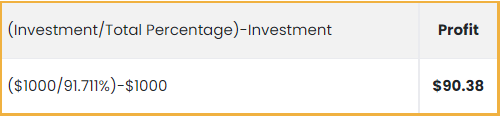## Calculating individual bets

Finally, you have to calculate individual bets you have to place to make the same profit no matter who wins. In order to get individual bets you have to multiply investment with individual percentage and then divide it with total arbitrage percentage. The formula is: individual bet = (investment * individual percentage)/ total arbitrage percentage.

Calculating Individual Bets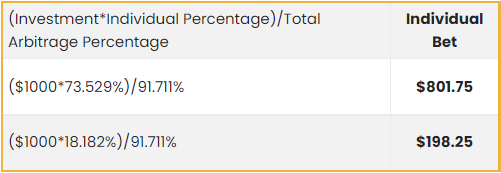So for this example you have to place \$801.75 on Djokovic and the remaining \$198.25 on Nadal in order to make \$90.38 profit of a \$1000 investment.

If you think this is too much maths, do not worry since all these calculations can be done in few seconds with arbitrage calculator. This page has a purpose to explain you how sports arbitrage works. Every day you can find hundreds of arbitrage bets with profit between 1-5% and a couple of bets with profit above 15%. If you want to achieve maximum profit and avoid any possible mistakes read arbitrage advice.